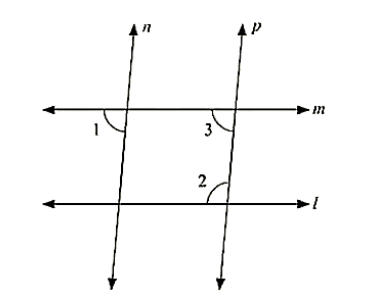# In the below fig. if l ∥ m, n ∥ p and ∠1 = 85°. find ∠2.

Question:

In the below fig. if l ∥ m, n ∥ p and ∠1 = 85°. find ∠2.Solution:

Corresponding angles are equal

⟹ ∠1 = ∠3 = 85°

By using the property of co-interior angles are supplementary

∠2 + ∠3 = 180°

∠2 + 55 = 180

∠2 = 180 − 85

∠2 = 95°